The non-Markovian nature of turbulence 7: non-Markovian closures and the LET theory in particular.

We can sum up the situation regarding the failure of the pioneering closures as follows. Their form of the transfer spectrum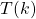, with its division into input and output parts, with the latter being proportional to the amount of energy in mode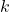, is only valid for Markov processes, so it is incompatible with the nature of turbulence which is non-Markovian. It is also incompatible with the phenomenology of turbulence, where the entireacts as input (or output), depending on the value of, as I pointed out in 1974 . It is worth noting that the first measurement ofwas made by Uberoi in 1963 , so turbulence phenomenology was in its infancy at the time the first closures were being developed. In later years, numerical experiments based on high-resolution direct numerical simulations, did not bear out the Markovian picture. In particular, we note the investigation by Kuczaj et al . This is in fact the basic flaw in Kraichnan’s DIA and also the SCF theories of Edwards and Herring: the fault lies not in the covariance equations but in the relationship of the response function to them.

As mentioned in the first blog in the present series (posted on 13 October) a response to this problem took, and continues to take, the form of an extension of the DIA approach to Lagrangian coordinates. A consideration of these theories would take us too far away from our present objective although it should be mentioned that they are non-Markovian in that they are not expressible as Master equations. Instead we will concentrate on the LET theory which exposes the underlying physics of the turbulence energy transfer process.

The LET theory was introduced with the hypothesis that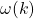is determined by the entire, not just part of it, and can be defined by a local energy balance . It was extended to the two-time case  in 1978; and, less heuristically, in subsequent papers by McComb and co-workers: see  for a review. Essentially, the two-time LET theory comprises the DIA covariance equations plus the generalized fluctuation-response relation. It may be compared to Herring’s two-time SCF  which comprises the DIA response equation, single-time covariance equation and the generalized fluctuation-response equation. It may also be compared directly to DIA in terms of response equations. However, for our present purposes, we will go back to the simplest case, and show how LET arose in relation to the Edwards SCF.

It was argued by McComb , that a correct assignment of the system response in terms of(i.e. `correct’ in the sense of agreeing with the turbulence phenomenology of energy transfer) could lead to a response function which was compatible with K41. This was found to be the case and, citing the form given in , we may write for the turbulence viscosity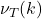:

(1)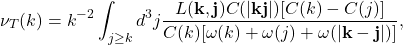where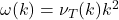. The lower limit on the integral with respect to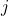arises when we consider the flux through mode. It was used in  to justify wavenumber expansions leading to differential forms but is not needed here and can be omitted. The interesting point here is made by rewriting this in terms of the Edwards dynamical friction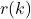. From equation (5) in the post on 3 November, rewritten as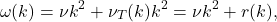we may rewrite (1) as:

(2)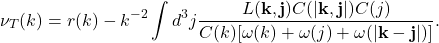It was shown  that the second term in the LET  response equation  cancelled the divergence inin the limit of infinite Reynolds number. Hence the term which destroys the Markovian nature of the renormalized perturbation theory is the term which makes the theory compatible with the Kolmogorov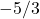spectrum.

In the next post we will consider the subject of almost-Markovian models, where the term refers to the integrals over time rather than to the energy transfer through wavenumber.

 W. D. McComb. A local energy transfer theory of isotropic turbulence. J. Phys. A, 7(5): 632, 1974.
 M. S. Uberoi. Energy transfer in isotropic turbulence. Phys. Fluids, 6:1048, 1963.
 Arkadiusz K. Kuczaj, Bernard J. Geurts, and W. David McComb. Nonlocal modulation of the energy cascade in broadband-forced turbulence. Phys. Rev. E, 74:16306-16313, 2006.
 W. D. McComb. A theory of time dependent, isotropic turbulence. J. Phys. A: Math. Gen., 11(3):613, 1978.
 W. D. McComb and S. R. Yoffe. A formal derivation of the local energy transfer (LET) theory of homogeneous turbulence. J. Phys. A: Math. Theor., 50:375501, 2017.
 J. R. Herring. Self-consistent field approach to nonstationary turbulence. Phys. Fluids, 9:2106, 1966.